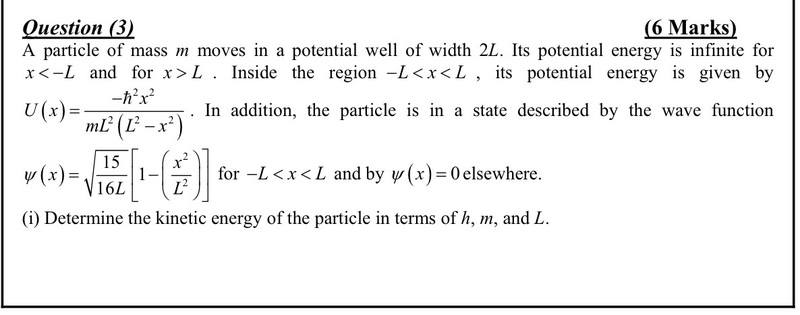# Quantum mechanics problem

## Homework Statement## Homework Equations

Time independent schrodinger equation

## The Attempt at a Solution

I interpretted the schrodinger equation as kinetic energy plus potential energy equals total energy, but i am not sure this makes much sense